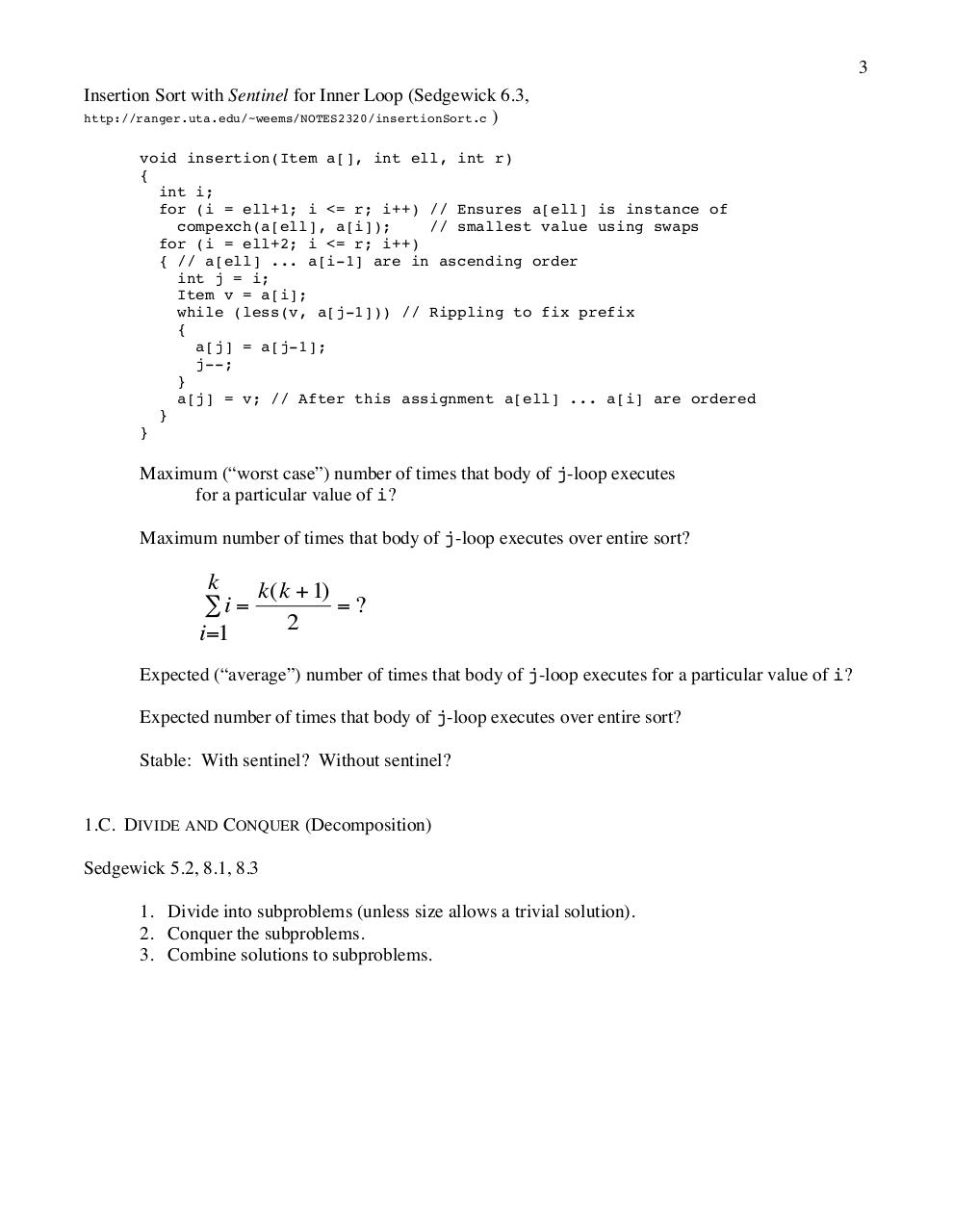# notes01.pdfPage 1 2 3 4 5 6 7 8 9 10

#### Text preview

3
Insertion Sort with Sentinel for Inner Loop (Sedgewick 6.3,
http://ranger.uta.edu/~weems/NOTES2320/insertionSort.c )
void insertion(Item a[], int ell, int r)
{
int i;
for (i = ell+1; i &lt;= r; i++) // Ensures a[ell] is instance of
compexch(a[ell], a[i]);
// smallest value using swaps
for (i = ell+2; i &lt;= r; i++)
{ // a[ell] ... a[i-1] are in ascending order
int j = i;
Item v = a[i];
while (less(v, a[j-1])) // Rippling to fix prefix
{
a[j] = a[j-1];
j--;
}
a[j] = v; // After this assignment a[ell] ... a[i] are ordered
}
}

Maximum (“worst case”) number of times that body of j-loop executes
for a particular value of i?
Maximum number of times that body of j-loop executes over entire sort?

k
k(k + 1)
=?
∑i =
2
i=1
Expected (“average”) number of times that body of j-loop executes for a particular value of i?

Expected number of times that body of j-loop executes over entire sort?
Stable: With sentinel? Without sentinel?

1.C. DIVIDE AND CONQUER (Decomposition)
Sedgewick 5.2, 8.1, 8.3
1. Divide into subproblems (unless size allows a trivial solution).
2. Conquer the subproblems.
3. Combine solutions to subproblems.